Thermodynamics and Propulsion

# 15.4 First Law Analysis of Reacting Systems

The form of the first law for the control volume is (there is no shaft work):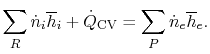This is given in terms of the moles of the different constituents, and it reduces to the more familiar form for a single fluid (say air) with no reactions occurring. We need to specify one parameter as the basis of the solution; 1 kmole of fuel, 1 kmole of air, 1 kmole total, etc. We use 1 kmole of fuel as the basic unit and examine the burning of hydrogen.The reactants and the products are both taken to be atand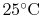, so the inlet and exit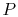andare specified. The control volume is the combustion chamber. There is no shaft work done and the SFEE is in the form of Equation (15.2). The enthalpy of the entering gas is zero for both the hydrogen and the oxygen (elements have enthalpies defined as zero at the reference state). If the exit products are in the gaseous state, the exit enthalpy is therefore related to the enthalpy of formation of the product by: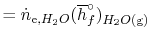If the water is in a liquid state at the exit of the process: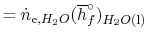There is more heat given up if the products emerge as liquid. The difference between the two values is the enthalpy needed to turn the liquid into gas at:.

A more complex example is provided by the burning of methane (natural gas) in oxygen, producing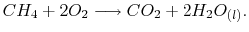The components in this reaction equation are three ideal gases (methane, oxygen, and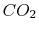) and liquid water. We again specify that the inlet and exit states are at the reference conditions so that: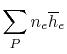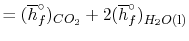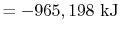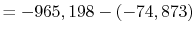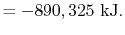Suppose the substances which comprise the reactants and the products are not atand. If so, the expression that connects the reactants and products is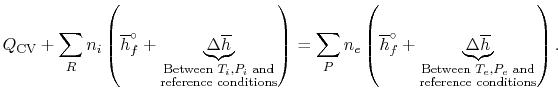(15..4)

Equation (15.4) shows that we must compute the enthalpy difference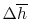between the reference conditions and the given state if the inlet or exit conditions are not the reference pressure and temperature.

There are different levels of approximation for the computation:

1. Assume the specific heat is constant over the range at some average value,
2. Use the polynomial expressions (Table A.6) in the integral,
3. Use tabulated values.
The first is the simplest and the crudest. Combustion processes often involve changes of a thousand degrees or more and, as Figure 15.2 shows, the specific heat for some gases can change by a factor of two or more over this range, although the changes for air are more modest. This means that, depending on the accuracy desired, one may need to consider the temperature dependence of the specific heat in computing.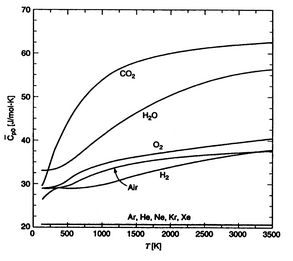Muddy Points

When doing cycle analysis, do we have to consider combustion products and their effect on specific heat ratio (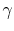is not 1.4)? (MP 15.6)

UnifiedTP